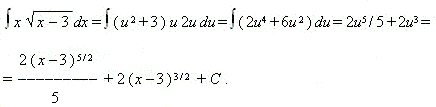# Integration methods

Integration by parts. Integration by substitution ( exchange ).

Integration by parts. If functions u ( x ) and v ( x ) have continuous first derivatives and the integral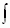v ( x ) du ( x ) exists, then the integralu ( x ) dv ( x ) also exists and the equalityu ( x ) dv ( x ) = u ( x )

v ( x ) –v ( x ) du ( x )

takes place, or shortly:u dv = u vv du .

Pay attention to the fact, that this operation and a differential of product of two functions are mutually inverse operations ( check, please ! ).

 E x a m p l e. Find the integral:ln x dx . S o l u t i o n. If to suppose that u = ln x and dv = dx , then du = dx / x and v = x . Using the formula of integration by parts, we receive: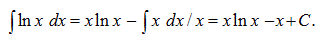Integration by substitution (exchange). If a function f ( z ) is given and has a primitive at z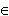Z , a function z = g ( x ) has a continuous derivative at xX , and g ( X )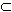Z , then the function F ( x ) = f [ g ( x )]

g' ( x ) has a primitive in Õ andF ( x ) dx =f [ g ( x )]

g' ( x ) dx =f ( z ) dz .

 E x a m p l e. Find the integral: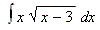. S o l u t i o n. To get rid of the square root we assume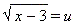, then x = u 2 + 3 and hence, dx = 2 u du . Then exchanging, we have: